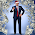## Thursday, June 11, 2015

### Proton Decay - A Work In Progress

Delicate flowers decay
(but into what? Bions)
.
$\dots$
$\vdots$
$$M_PR_P=4LM$$
$${\partial{(M_PR_P)}\over{\partial t}}={\partial{(4LM)}\over\partial t}$$
$${\partial{(4LM)}\over\partial t}=0$$

$${\partial{(M_PR_P)}\over{\partial t}}={R_P\partial{M_P}+M_P\partial{R_P}\over\partial t}=0$$
$${R_P\partial{M_P}+M_P\partial{R_P}\over\partial t}=0$$
$$R_P\partial{M_P}=-{M_P\partial{R_P}}$$
$${\partial{M_P}\over M_P}=-{\partial R_P\over R_P}$$

$$ln(M_P)=-ln(R_P)+C$$
$$-ln(R_P)=ln({1\over R_P})$$
$$ln(M_P)=ln({1\over R_P})+C$$
$$M_P={e^C\over R_P}$$
$${M_PR_P}=K^{}$$
the mass.radius product of the proton is a constant $\therefore$ the proton does not decay.

 Also Lorentz transform invariant. Mass_in_special_relativity Length_contraction (ratios out)

There is no such thing as dark matter / dark energy - Rauscher-Haramein proved it with their extension to Einstein's field equations. There is no reason for the proton to decay, especially because a failed theory says it should decay to make everything consistent in those failed theories.

The BIG BANG has failed, SM has failed.  It is time to move on.

${\partial M_P\over\partial t}=0$ due to no known observable mass change of the proton at rest
${\partial R_P\over\partial t}=0$ due to no known observable radius change of the proton at rest

4,L, & M being constants and derived from measured constants, the partial or change or derivative of a constant with respect to anything is zero$^{}$.  We will look into varying these constants later as others have done (varying the cosmological constant, G, c, $\Lambda$, etc, in an appropriate way).
 except for wrt itself ${\partial K \over \partial K}=1$

Clearly, the proton mass.radius product is a constant.  It is a self-consistent, tautological, self-contained thing - the proton - a fractal representation of the whole AND compatible with our mathematics at this scale, at all scales. As above, so below.

Additional Supporting Derivations / proofs:  (add details and diagrams) TO BE ADDED later

1. Add diagram for proton + (antiproton + proton) = proton
2. Add summation of energy-mass, conservation of energy-mass-information
3. the proton vibrates into and out of the vacuum teleporting itself from place to place

Clearly the mainstream physics viewpoint of the proton is not adequate for describing its nature. Another viewpoint or concept is needed to describe the behaviour and nature of the proton.

The genesis of the proton - how it is/was created in the first place.  All of this has to be considered, as well as the future fate.

So, at this point, there are many proton structures to consider, many models to capture the protons essence, however, which one works to truly explain and predict the behavior of nature?

The wormhole connected proton of the unified physics...
(and there are a few other approaches to this problem we will be looking into)

So, if anyone has any comments, feel free...

Mainstream, for comparison:

http://en.wikipedia.org/wiki/Proton_decay

The Surfer, OM-IV
The Big Bang Theory - Proton Decay

#### 1 comment:

1.updated Omega link to Krusty

Watch the water. 🦆